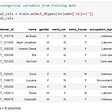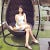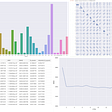# A Gentle Introduction to EDA(Exploratory Data Analysis) in Python

Exploratory Data Analysis, or EDA as it is commonly referred to is the process of analyzing your dataset. This comprises of the following three sections-

1. Understanding your data variables or columns
3. Analyzing relationships between variables or columns

We are going to use a popular dataset — iris for our use case here. Use this link to get it — iris.

1. df.shape returns the number of rows by the number of columns for my dataset.
`df.shape()(149, 5)`

2. df.head() returns the first 5 rows of my dataset.

`df.head()`

3. df.columns returns the name of all of your columns in the dataset.

`df.columnsIndex(['class', 'sepal length', 'sepal width', 'petal length', 'petal width'], dtype='object')`

4. df.nunique(axis=0) returns the number of unique values for each variable.

`df.nunique(axis=0)class            3sepal length    35sepal width     23petal length    43petal width     22dtype: int64`

5. df.describe() summarizes the count, mean, standard deviation, min, and max for numeric variables.

`df.describe`

1. Removing null values — This removes all the columns and rows that have null values. You can specify axis=0,1 if you want to restrict it to row or column only.
`df.dropna()`

2. Removing outliers —

Outliers can skew the data and lead to incorrect results. Hence, it is important to remove them. Here, we are using z-score to find the outliers.

`from scipy import statsimport numpy as npz = np.abs(stats.zscore(df[['sepal length','sepal width','petal length', 'petal width']]))threshold = 3 /*can be changed*/print(np.where(z > 3))(array(), array())`

This means row 15, column 1 data point is an outlier.

3. Removing redundant columns -

Sometimes more than one column can contain the same/similar value. In that case having two columns does not add any value to the model. So, it is wise to delete the redundant column.

4. Remove redundant rows -

This depends on the use case, but if having duplicate records does not make sense, then it is wise to remove the redundant rows -

`df.drop_duplicates()`

# Analyzing relationships between variables or columns

The final step is to determine any relations that exist between your data columns/variables.

1. Correlation Matrix

A correlation matrix is a table that shows the correlation coefficients between many variables.

`# calculate correlation matrixcorr = df.corr()# plot the heatmapimport seaborn as snssns.heatmap(corr, xticklabels=corr.columns, yticklabels=corr.columns, annot=True, cmap=sns.diverging_palette(220, 20, as_cmap=True))`

We can see from the matrix that there is positive correlation between sepal length and petal length, and negative correlation between sepal width and petal length.

2. Scatterplot

A Scatterplot is a type of graph which ‘plots’ the values of two variables along two axes, like age and height.

`df.plot(kind='scatter', x='sepal length', y='petal length')`

While there are many other plots such as histograms, box plots, pair plots etc.. you can explore for your use case, these cover the most common used EDA steps. Happy Analyzing !

--

--

--

## More from MLearning.ai

Data Scientists must think like an artist when finding a solution when creating a piece of code. ⚪️ Artists enjoy working on interesting problems, even if there is no obvious answer ⚪️ linktr.ee/mlearning 🔵 Follow to join our 18K+ Unique DAILY Readers 🟠

## Categorical variable encoding in Pandas## Drugs NER using spaCy in Python## Measuring What Makes Readers Subscribe to The New York Times## In addition to consumers and aggregators, Anderson also wrote about a third group:## On the 22nd May , 2020, I got a call from an unknown number, who declared that someone recommended…## Reading Surf Forecasts: Swell Height## Is there any relationship between the spread of the coronavirus in a country and how happy people…## Swagata Ashwani

I love talking Data! Data Scientist with a passion for finding optimized solutions in the AI space.Follow me here — https://www.linkedin.com/in/swagata-ashwani/

## Classification using Decision Trees## Price Prediction with Linear Regression## Choosing a Summary Statistic to Explore Datasets (Part 1 - Univariate)## Exploratory Data Analysis Using Python